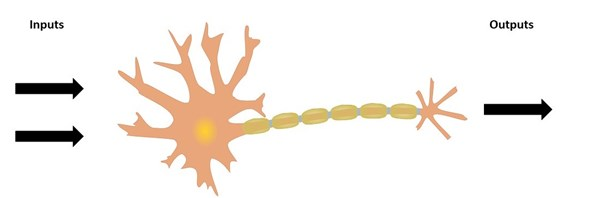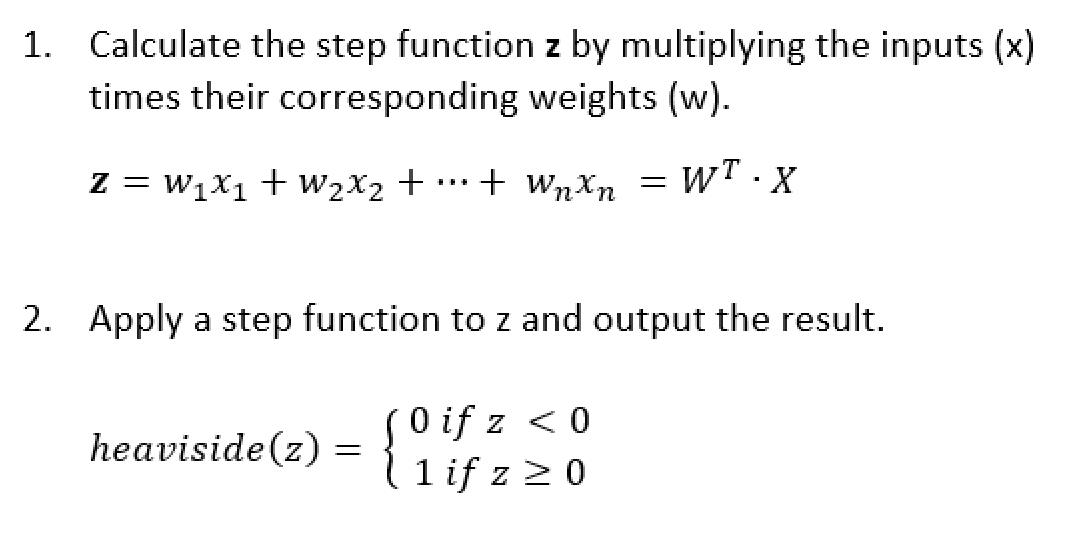Machine Learning

# Perceptron Algorithm Part 1 | Machine Learning 101

### What is it?

The perceptron is a supervised learning algorithm used for binary classification. It is one of the oldest machine learning algorithm dating back to the 1950’s and has been the inspiration to many state of the art algorithms used today. Learning how the perceptron algorithm works will give you the intuition to better understand more advanced Artificial Neural Network models such as Deep Learning.

### A bit of History

In essence, the perceptron is trying to model the the function of a single neuron cell. In 1943 Warren McCullock and Walter Pitts published “A logical Calculus of Ideas Immanent in Nervous Activity” in which they defined the concept of a simplified brain cell.

Frank Rosenblatt drew inspiration on this concept and invented the Perceptron learning rule in 1957. This algorithm has the capability to learn a set of weights which are used to determine if this “neuron” fires or not. This basic principle is used throughout Neural Networks model utilized today.### The Perceptron Algorithm

The perceptron uses the following algorithm to produce a binary output:The Heaviside Step function is the most common used in this algorithm, others such as the sign step function can also be used.

The perceptron is a binary classifier as can be seen by the two possible outputs from step 2. Note that the outputs are not probabilities.

### Training the Perceptron

The perceptron is trained by feeding it one training sample at a time and having it make a prediction. The algorithm will then use the result of this prediction to change its weights. The weights become the memory of the algorithm.

The steps are as follows:

1. Initialize all the weights to 0 or a small random number
2. For each training sample xi obtain the output value ẏ
3. Update the weights

To update the weights we will do the following: wj := wj + ∆wj

The value of ∆wj is obtained from the perceptron training rule:

∆wj = η(y(i) - ẏ(i)) xj(i)

Where:

• η = The learning rate which is typically a constant between 0 and 1.
• y(i) = the true class label of the ith training sample
• (i) = the predicted class label.

In the following post we will implement this algorithm in Python.#### MJ

Advanced analytics professional currently practicing in the healthcare sector. Passionate about Machine Learning, Operations Research and Programming. Enjoys the outdoors and extreme sports.

>